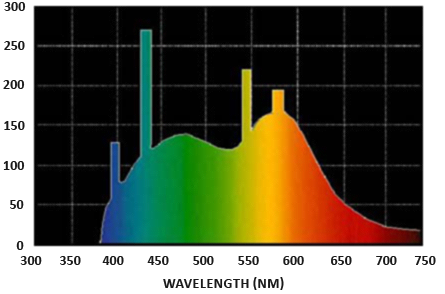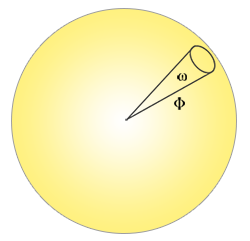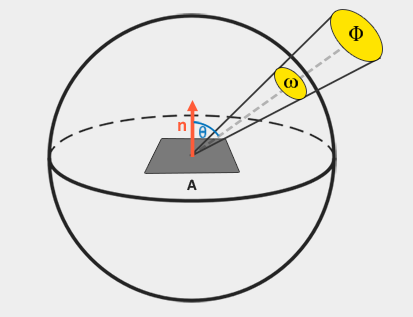# The Reflection Equation

## Overview

Physically based rendering strongly follows a more specialized version of the render equation known as the reflectance equation, which is the best model we have for simulating the visuals of light:

To understand the equation, we have to delve into a bit of radiometry. Radiometry is the measurement of electromagnetic radiation (including visible light). There are several radiometric quantities we can use to measure light over surfaces and directions, but we will only discuss a single one that’s relevant to the reflectance equation known as radiance, denoted here as $L$. Radiance is used to quantify the magnitude or strength of light coming from a single direction.

radiant flux $\Phi$ is the transmitted energy of a light source measured in Watts. Light is a collective sum of energy over multiple different wavelengths, each wavelength associated with a particular (visible) color. The emitted energy of a light source can therefore be thought of as a function of all its different wavelengths. Wavelengths between 390nm to 700nm (nanometers) are considered part of the visible light spectrum i.e. wavelengths the human eye is able to perceive. Below you’ll find an image of the different energies per wavelength of daylight:The radiant flux measures the total area of this function of different wavelengths. Directly taking this measure of wavelengths as input in computer graphics is slightly impractical so we often make the simplification of representing radiant flux not as a function of varying wavelength strengths, but as a light color triplet encoded as RGB This encoding does come at quite a loss of information, but this is generally negligible for visual aspects.

## Solid angle

the solid angle denoted as $\omega$ tells us the size or area of a shape projected onto a unit sphere. The area of the projected shape onto this unit sphere is known as the solid angle; you can visualize the solid angle as a direction with volume:radiant intensity measures the amount of radiant flux per solid angle or the strength of a light source over a projected area onto the unit sphere. For instance, given an omnidirectional light that radiates equally in all directions the radiant intensity can give us its energy over a specific area (solid angle):The equation to describe the radiant intensity is defined as follows:

Where $I$ is the radiant flux $\Phi$ over the solid angle $\omega$.

With knowledge of radiant flux, radiant intensity and the solid angle we can finally describe the equation for radiance, which is described as the total observed energy over an area $A$ over the solid angle $\omega$ of a light of radiant intensity $\Phi$:Radiance is a radiometric measure of the amount of light in an area scaled by the incident (or incoming) angle $\theta$ of the light to the surface’s normal as $cos\theta$: light is weaker the less it directly radiates onto the surface and strongest when it is directly perpendicular to the surface. This is similar to our perception of diffuse lighting from the basic lighting tutorials as $cos\theta$ directly corresponds to the dot product between the light’s direction vector and the surface’s normal:

## The Reflection Equation

The radiance equation is quite useful as it consists of most physical quantities we’re interested in. If we consider the solid angle ω and the area A to be infinitely small, we can use radiance to measure the flux of a single ray of light hitting a single point in space. This relation allows us to calculate the radiance of a single light ray influencing a single (fragment) point; we effectively translate the solid angle ω into a direction vector ω, and A into a point p. This way we can directly use radiance in our shaders to calculate a single light ray’s per-fragment contribution.

In fact, when it comes to radiance we generally care about all incoming light onto a point p which is the sum of all radiance known as irradiance. With knowledge of both radiance and irradiance we can get back to the reflectance equation:

We now know that $L$ in the render equation represents the radiance of some point p and some incoming infinitely small solid angle $\omega_i$ which can be thought of as an incoming direction vector $\omega_i$. Remember that $cos\theta$ scales the energy based on the light’s incident angle to the surface which we find in the reflectance equation as $n\cdot \omega_o$. The reflectance equation calculates the sum of reflected radiance $L_o(p,\omega_o)$ of a point $p$ in direction $\omega_o$ which is the outgoing direction to the viewer. Or to put it differently: $Lo$ measures the reflected sum of the lights’ irradiance onto point $p$ as viewed from $\omega_o$.

As the reflectance equation is based around irradiance which is the sum of all incoming radiance we measure light of not just a single incoming light direction, but of all incoming light directions within a hemisphere $\Omega$ centered around point $p$. A hemisphere can be described as half a sphere aligned around a surface’s normal $n$:To calculate the total of values inside an area or, in the case of a hemisphere, a volume we use a mathematical construct called an integral denoted in the reflectance equation as $\int$ over all incoming directions $d\omega_i$ within the hemisphere $\Omega$. An integral measures the area of a function, which can either be calculated analytically or numerically. As there is no analytical solution to both the render and reflectance equation we’ll want to numerically solve the integral discretely. This translates to taking the result of small discrete steps of the reflectance equation over the hemisphere $\Omega$ and averaging their results over the step size.

The reflectance equation sums up the radiance of all incoming light directions $\omega_i$ over the hemisphere $\Omega$ scaled by $f_r$ that hit point $p$ and returns the sum of reflected light $L_o$ in the viewer’s direction. The incoming radiance can come from light sources.

Now the only unknown left is the $f_r$ symbol known as the BRDF or Bidirectional Reflective Distribution Function that scales or weighs the incoming radiance based on the surface’s material properties.

## Reference

Learn OpenGL PBR Theory

Share